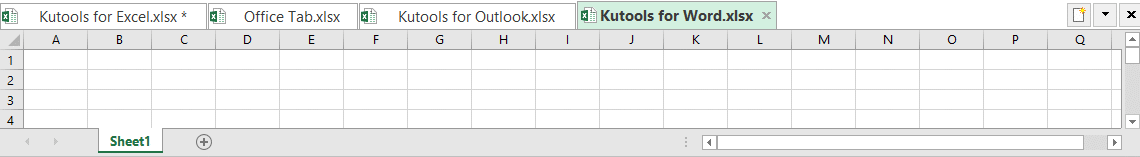## Excel add text and number into specified position of cell

In Excel, adding texts or numbers to cells is a very common job. Such as adding space between names, adding prefix or suffix to cells, adding dashes to social numbers. Here in this tutorial, it lists almost all of adding scenarios in Excel and provides the corresponding methods for you.

Note

In this tutorial, I create some examples to explain the methods, you can change the references for you need when you use below VBA code or formulas, or you can download the samples for trying methods directly.Click to download the sample file

#### 1. Add to start of each cell

This part lists different methods on adding characters to the start of each cell as below screenshot shown: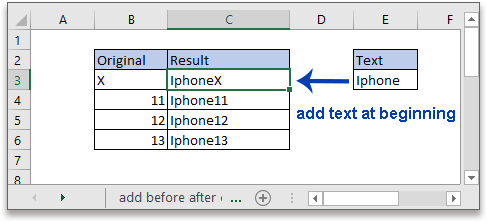1.1 Add at beginning of cells by using formula

Here you can choose one of below three formulas:

Formula1 Concatenate operator "&"

Join multiple texts together by an ampersand character "&".

"text"&cell

Formula2 CONCATENATE function

CONCATENATE function is used to join texts together.

CONCATENATE("text",cell)

Formula3 CONCAT function

This is a new function which only appears in Excel 2019, Office 365, and Excel online.

CONCAT("text",cell)

In the generic formulas: cell is the cell reference to the text that you want to add prefix to, and text is the text that used to add to the cell.

Using the example above, you can use the formulas in formula bar as these:

"&"

=\$E\$3&B3 or ="Iphone"&B3

CONCATENATE function

=CONCATENATE(\$E\$3,B3) or =CONCATENATE("Iphone",B3)

CONCAT function

=CONCAT(\$E\$3,B3) or =CONCAT("Iphone",B3)

Press Enter key to get the result, then drag autofill handle down to add text to each cell of range B3:B6.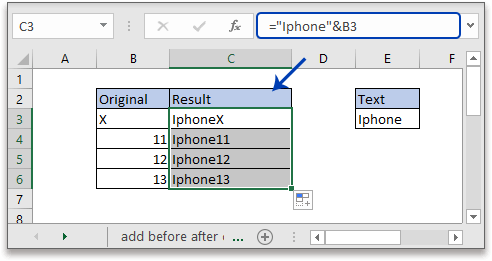Attention: Text should be enclosed in double quotes, or formula returns an error value #NAME?. If using the cell reference to a text, please remember to use the absolute reference, you can press F4 key to change the relative reference to absolute reference.

1.2 Add at beginning of cells by Flash Fill

If you are in Excel 2013 or later versions, the powerful new feature, Flash Fill, can fill the cells based on the above cells you entered automatically.

Choose a cell next to the first original data, manually type the first data with the prefix text as below shown: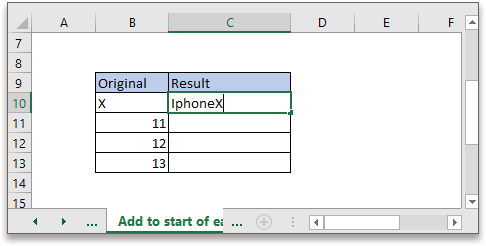Then in cell below, continue typing the second data with prefix text，during the typing, a list in grey color will display, press Enter key to allow the Flash Fill to fill the data automatically.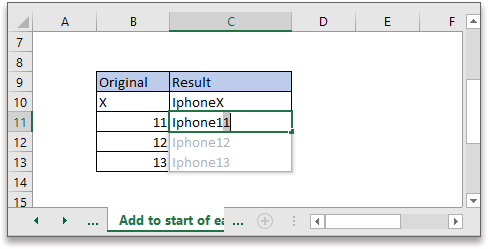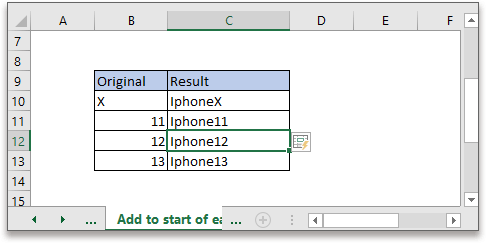If the preview list does not generate, please go to the Home tab, and then click Fill > Flash Fill to run it manually.

1.3 Add at beginning of cells by using VBA code

If you are familiar with VBA code, here introduces a VBA code to add text at beginning of each cell in a range.

1. Select a range of cells that you want to add the prefix text, then press Alt + F11 keys to enable the Microsoft Visual Basic for Applications window.

2. Then in the popping window, click Insert > Module to insert a blank new module.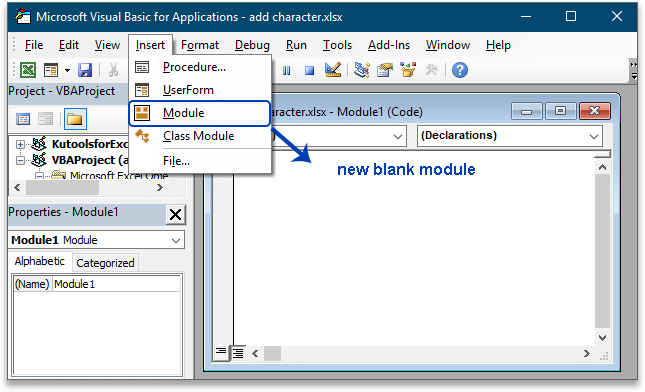3. Copy and paste below VBA code to the new module.

VBA: Add at beginning of cells

``````Sub AppendToExistingOnLeft()
Dim c As Range
For Each c In Selection
If c.Value <> "" Then c.Value = "Iphone" & c.Value
Next
End Sub``````
 Attention: in the code script, Iphone is the text you want to add at beginning of selected cells, change it for your need.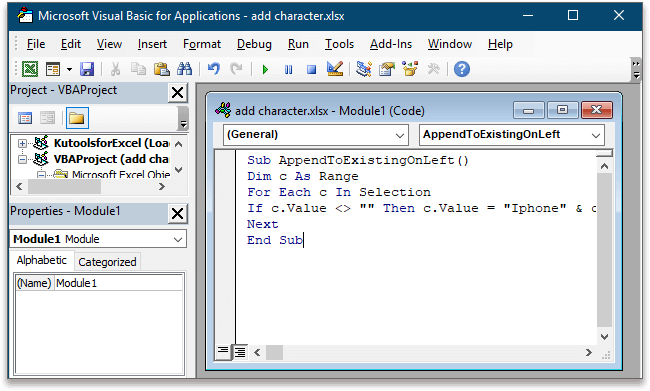4. Press F5 key or click Run button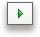in the window to activate the VBA code.

Now all selected cells have been added the text "Iphone" at the beginning.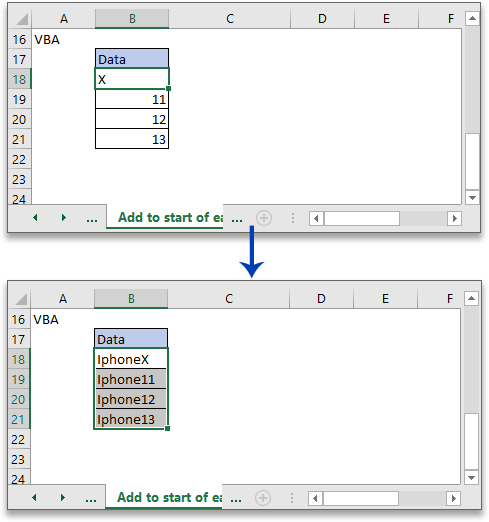#### 2. Add to end of each cell

Except adding text to start of cells, adding text to end of cells is also common. Here we take adding unit at end of each cell for examples as below screenshot shown: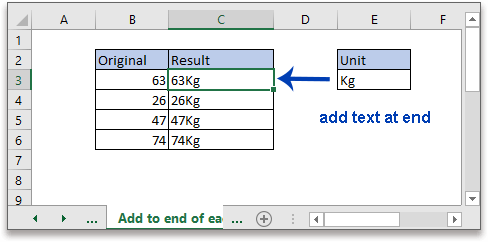2.1 Add at end of cells by using formula

Here you can choose one of below three formulas:

Formula1 Concatenate operator "&"

Join multiple texts together by an ampersand character "&".

Cell&"text"

Formula2 CONCATENATE function

CONCATENATE function is used to join texts together.

CONCATENATE(cell,"text")

Formula3 CONCAT function

This is a new function which only appears in Excel 2019, Office 365 and Excel online.

CONCAT(cell,"text")

In the generic formulas: cell is the cell reference to the text that you want to add suffix to, and text is the text that used to add to the cell.

Using the example above, you can use the formulas in formula bar as these:

"&"

=B3&\$E\$3 or =B3&"Kg"

CONCATENATE function

=CONCATENATE(B3, \$E\$3) or =CONCATENATE(B3, "Kg")

CONCAT function

=CONCAT(B3, \$E\$3) or =CONCAT(B3, "Kg")

Press Enter key to get the result, then drag autofill handle down to add text to the end of each cell of range B3:B6.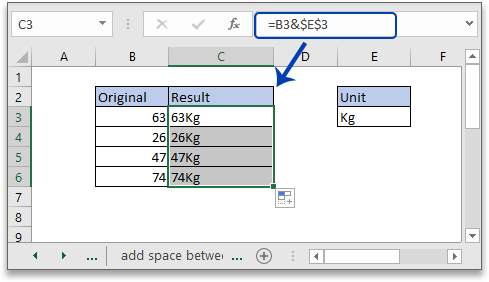Attention: Text should be enclosed in double quotes, or formula returns an error value #NAME?. If using the cell reference to a text, please remember to use the absolute reference, you can press F4 key to change the relative reference to absolute reference.

2.2 Add at end of cells by Flash Fill

If you are in Excel 2013 or later versions, the powerful new feature, Flash Fill, can fill the cells based on the above cells you entered automatically.

Choose a cell next to the first original data, manually type the first data with the suffix text as below shown: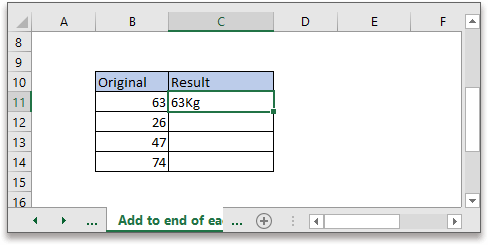Then activate the cell under the first result, click Data > Flash Fill, all below cells including the active cell will be filled automatically.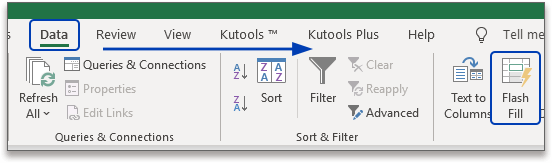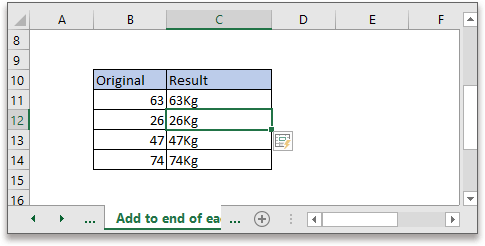2.3 Add at end of cells by using VBA code

Here is a VBA code can add text at end of each cell in a range.

1. Select a range of cells that you want to add the suffix text, then press Alt + F11 keys to enable the Microsoft Visual Basic for Applications window.

2. Then in the popping window, click Insert > Module to insert a blank new module.3. Copy and paste below VBA code to the new module.

VBA: Add at end of cells

``````Sub AppendToExistingOnRight()
Dim c As Range
For Each c In Selection
If c.Value <> "" Then c.Value = c.Value & "Kg"
Next
End Sub``````
 Attention: in the code script, Kg is the text you want to add at end of selected cells, change it for your need.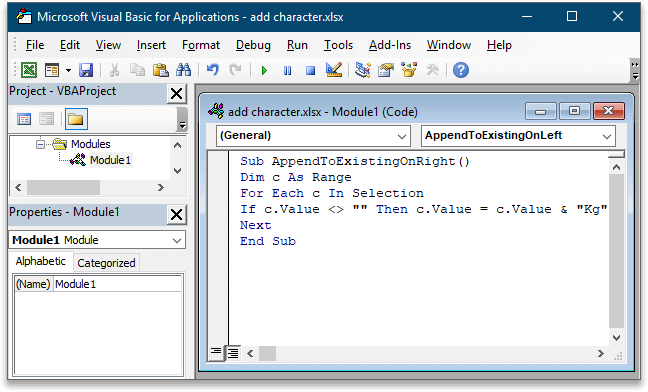4. Press F5 key or click Run buttonin the window to activate the VBA code.

Now the text "Kg" has been added at end of each cell.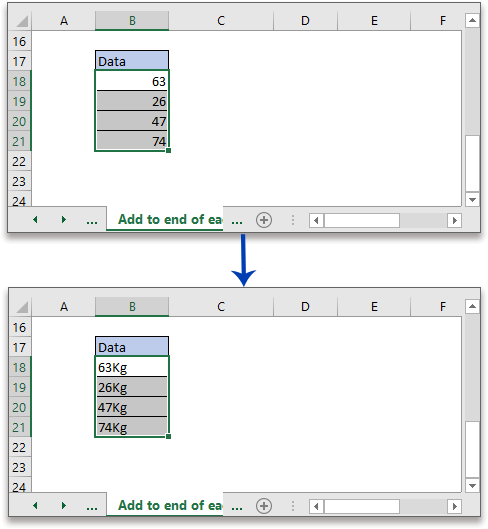#### 3. Add to middle of string

For most of you, adding texts to the start or end of cells are easy, but adding texts to middle of cells maybe is somewhat difficult. Take instance, as below screenshot shown, adding a delimiter, such as colon : at the end of the second character of each cell in range B3:B7.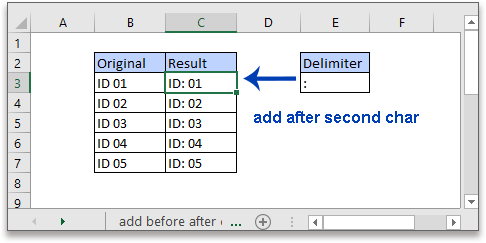3.1 Add after nth character of string by formula

Here are four formulas provided for you to handle this job, choose one to use:

Formula1 Combine LEFT and RIGHT functions

LEFT(cell, n) & "text" & RIGHT(cell, LEN(cell) -n)

Formula2 COMBINE CONCATENATE(CONCAT), RIGHT and LEN functions

CONCATENATE(LEFT(cell, n), "text", RIGHT(cell, LEN(cell) -n))

Or

CONCAT(LEFT(cell, n), "text", RIGHT(cell, LEN(cell) -n))

Formula3 REPLACE function

REPLACE(cell, n+1, 0, "text")

In the generic formulas: cell is the cell reference to the text that you want to add text at middle, and text is the text that used to add to the cell, n is the number defines after which character in the text string you want to add text.

For solving the job mentioned above, please use the formulas as these:

Combine LEFT and RIGHT functions

=LEFT(B3, 2) & ":" & RIGHT(B3, LEN(B3) -2) or =LEFT(B3, 2) & \$E\$3 & RIGHT(B3, LEN(B3) -2)

COMBINE CONCATENATE(CONCAT), RIGHT and LEN functions

=CONCATENATE(LEFT(B3, 2), ":", RIGHT(B3, LEN(B3) -2)) or =CONCAT (LEFT(B3, 2), ":", RIGHT(B3, LEN(B3) -2))

REPLACE function

=REPLACE(B3, 2+1, 0, ":") or =REPLACE(B3, 2+1, 0, \$E\$3)

Press Enter key to get the result, then drag autofill handle down to add text after second character of each cell of range B3:B7.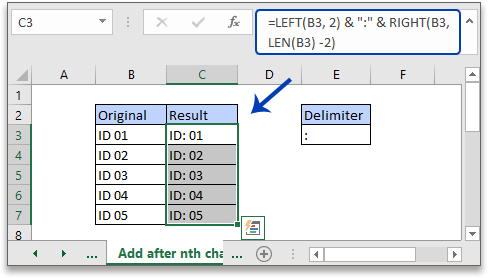Attention: Text should be enclosed in double quotes, or formula returns an error value #NAME?. If using the cell reference to a text, please remember to use the absolute reference, you can press F4 key to change the relative reference to absolute reference.

3.2 Add after nth character of string by Flash Fill

If you are in Excel 2013 or later versions, the Flash Fill, can fill the cells based on the above cells you entered automatically.

Choose a cell next to the first original data, manually type the first data with a colon after the second character as below shown: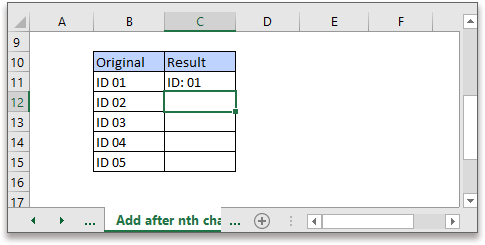Then activate the cell under the first result, press Ctrl + E keys to activate Flash Fill command, all below cells including the active cell have been filled automatically.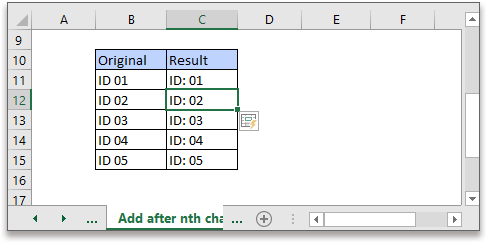Attention: If you are in MAC system, press Command + E keys.

3.3 Add after nth character of string by using VBA code

A VBA code can do this job, too.

1. Press Alt + F11 keys to enable the Microsoft Visual Basic for Applications window.

2. Then in the popping window, click Insert > Module to insert a blank new module.3. Copy and paste below VBA code to the new module.

VBA: Add to middle of cells

``````Sub AddToMidduleOfString()
Dim Rng As Range
Dim WorkRng As Range
On Error Resume Next
xTitleId = "KutoolsforExcel"
Set WorkRng = Application.Selection
Set WorkRng = Application.InputBox("Range", xTitleId, WorkRng.Address, Type:=8)
For Each Rng In WorkRng
Rng.Value = VBA.Left(Rng.Value, 2 & ":" & VBA.Mid(Rng.Value, 3, VBA.Len(Rng.Value) - 1)
Next
End Sub``````
 Attention: in the code script, 2 is the number defines the position that you want to add text after, : is the text you want to add to, 3 is the sum of 2 and the length of text you add. Take another instance, to add "and" after first character of string "A B", the script should be Rng.Value = VBA.Left(Rng.Value, 1) & "and" & VBA.Mid(Rng.Value, 4, VBA.Len(Rng.Value) - 1)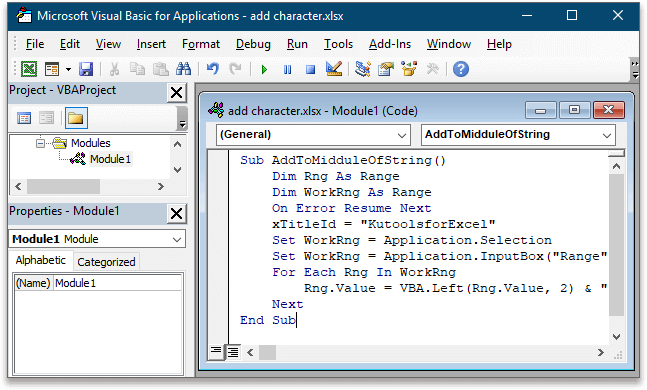4. Press F5 key or click Run buttonin the window to activate the VBA code. A dialog pops out for selecting cells to add text.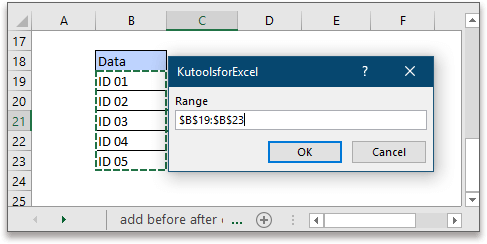5. Click OK. Now the text ":" has been added after the second character of each cell in range B19:B23.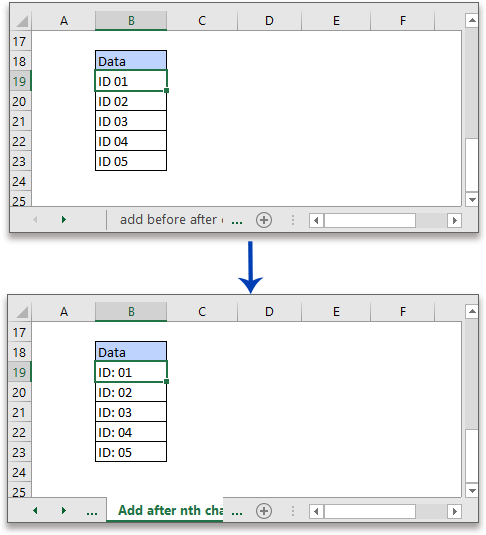#### 4. Add text with Kutools for Excel (add characters to specific position as you need)

If you are tired of formulas and VBA, you can try a powerful and handy tool, Kutools for Excel, which can help you with its Add Text feature.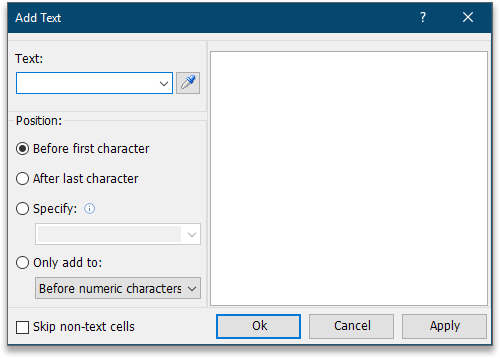• Add text before first character
• Add text after last character
• Add text at specified positions
• Extension

Click to get 30-day free trial now.

Select the cells that you want to add text, then apply the Add Text by clicking Kutools > Text > Add Text.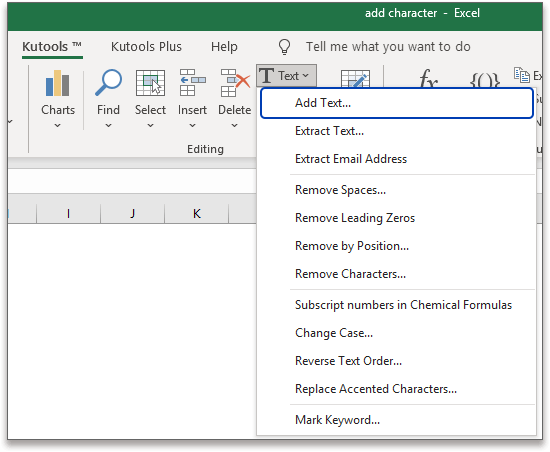4.1 Add text before first character or after last character

In the Add Text dialog, type the text that you want to add to cells in the Text textbox, then check Before first character in the Position section.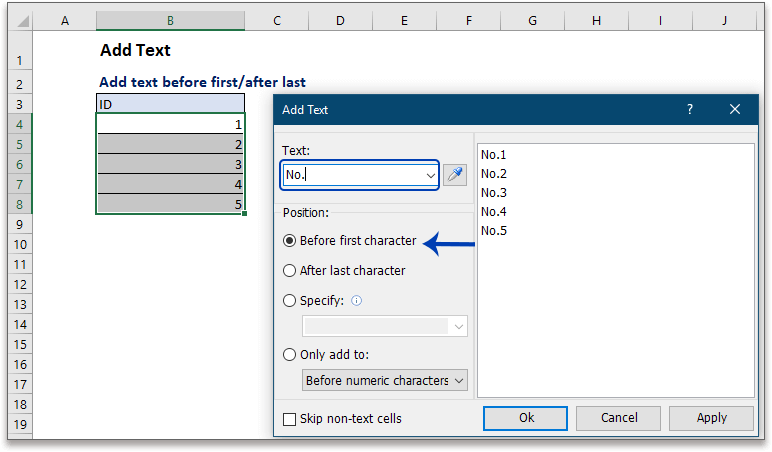Click Apply or Ok to finish the adding.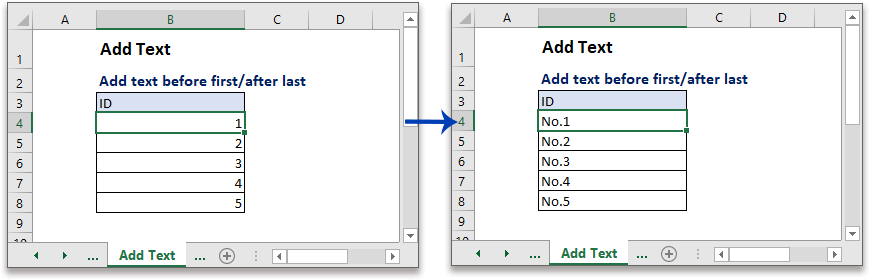To add text after last character, type the text that you want to add to cells in the Text textbox, then check After last character in the Position section.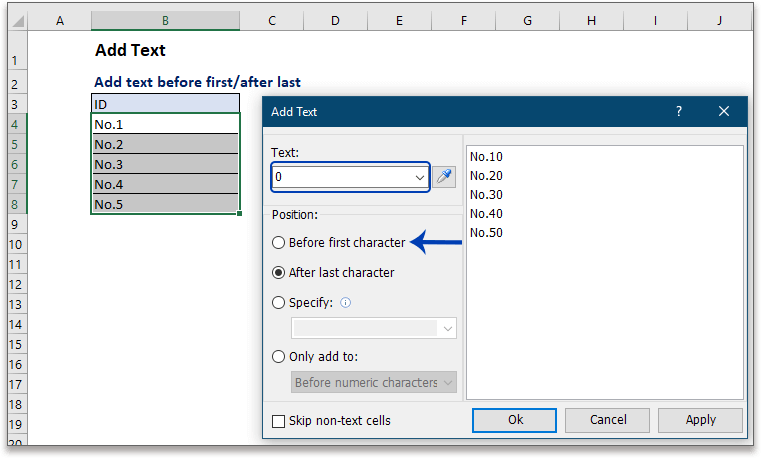Click Apply or Ok to finish the adding.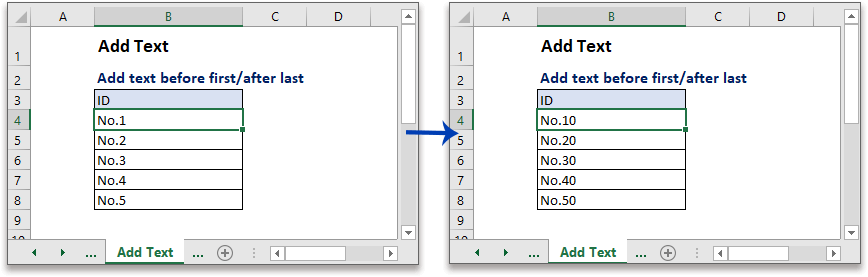4.2 Add text at specified position(s)

Sometimes, you may want to add text to the middle of cell, or add text to several positions of the cell. Supposing adding separator "-" after the third and seventh digits of the social numbers as below screenshot shown: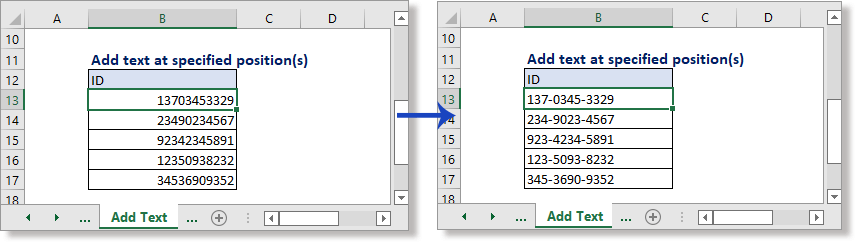In the Add Text dialog, type the text into Text textbox, and check Specify option, then type the positions using comma to separate into the textbox and then click Ok or Apply.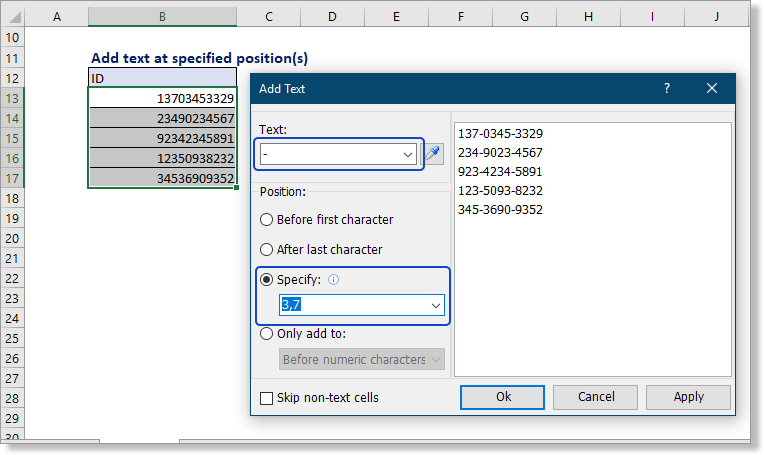4.3 Extension of Add Text utility

Beside adding text into the normal positions (beginning, end and specified position), the Add Text utility also supports to add text

• Before uppercase letters
• Before lowercase letters
• Before uppercase/lowercase letters
• Before numeric characters

In the Add Text dialog, type the text into Text textbox, and check Only add to option, then click at the drop-down menu below to choose the operation as you need.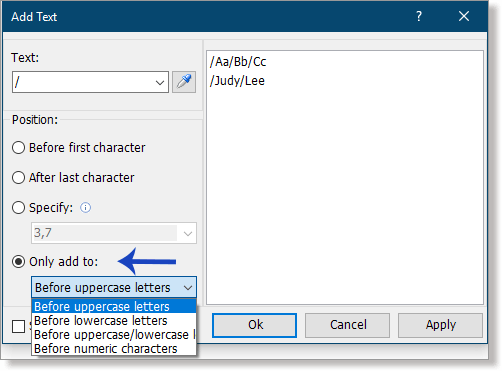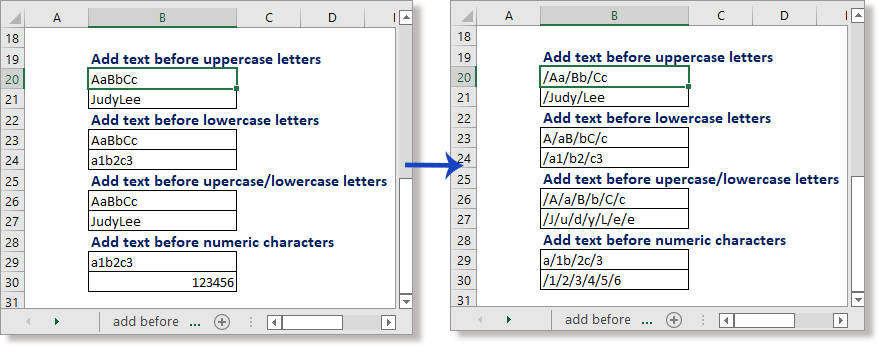Except Add Text feature, Kutools for Excel has other 300+ useful features which can do you a favor in Excel different jobs, get the free download now.

#### 5. Add text before or after the first specific text

This part provides formulas on adding text before or after the first appeared specific text in Excel.

5.1 Add text before the first specific text

For example, you want to add text "(sale)" before the first text "price" in the cell as below screenshots shown: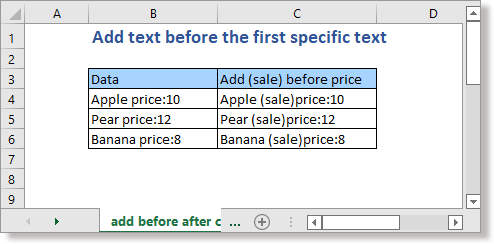In Excel, the REPLACE and SUBSTITUTE functions can solve this job.

REPLACE function

SUBSTITUTE function

SUBSTITUTE(cell,"find_text","replace_text",1)

In this case, please use the above formulas as these:

=REPLACE(B4,FIND("price",B4),0,"(sale)")

Or

=SUBSTITUTE(B4,"price","(sale)price",1)

The formula arguments in this case are

Cell: B4,

Find_text: price,

Replace_text:(sale)price.

Press Enter key to get the first adding result, then drag autofill handle over cells that need this formula.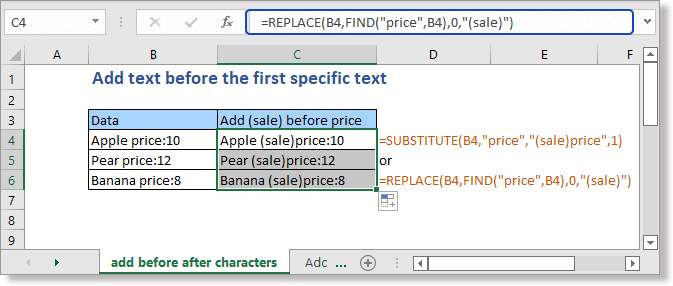5.2 Add text after the first specific text

For example, you want to add currency symbol "\$" after the first colon ":" in the cell as below screenshots shown: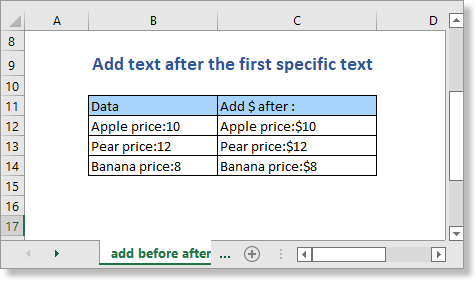In Excel, the REPLACE and SUBSTITUTE functions can solve this job.

REPLACE function

REPLACE(cell,FIND("find_text",cell),find_text_length,"replace_text")

SUBSTITUTE function

SUBSTITUTE(cell,"find_text","replace_text")

In this case, please use the above formulas as these:

=REPLACE(B12,FIND(":",B12),1,":\$")

Or

=SUBSTITUTE(B12,":",":\$")

The formula arguments in this case are

Cell: B12,

Find_text: :,

Replace_text::\$.

Find_text_length:1

Press Enter key to get the first adding result, then drag autofill handle over cells that need this formula.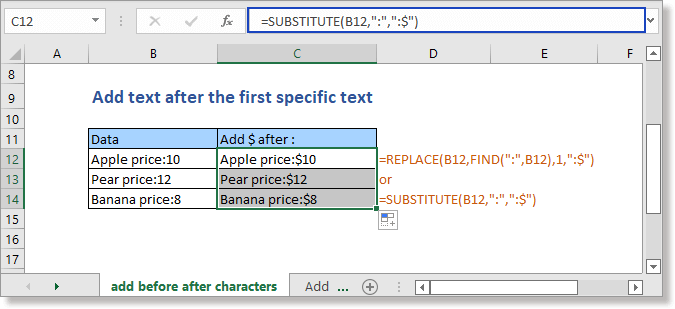#### 6. Add character(s) before or after each word

In this part of the tutorial, it introduces three different methods (formula, Find and Replace, VBA) to help you add characters before or after each word of cell.

 Add characters before each word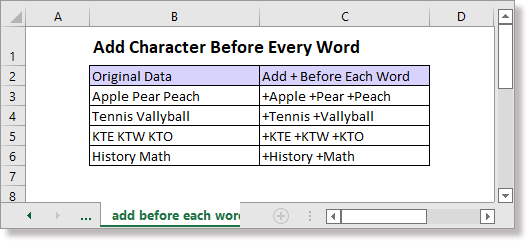Add characters after each word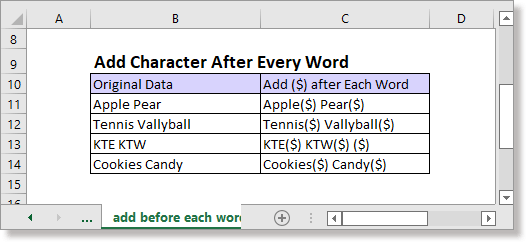6.1 Add character(s) before or after each word by formula

The SUBSTITUTE function can be used to solve this job, the general formulas as these:

"character"&SUBSTITUTE(string, " "," character")

SUBSTITUTE(string," ","character ")&"character"

Supposing adding "+" before every word in the list of B3:B6, please use the formula as this:

="+"&SUBSTITUTE(B3, " "," +")

Press Enter key to get the first result, then drag auto fill handle down to get all results.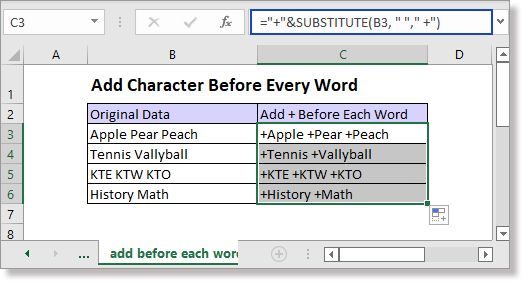Supposing adding (\$) after each word of list B11:B14, please use the formula as below:

=SUBSTITUTE(B11," ","(\$) ")&"(\$)"

Press Enter key to get the first result, then drag auto fill handle down to get all results.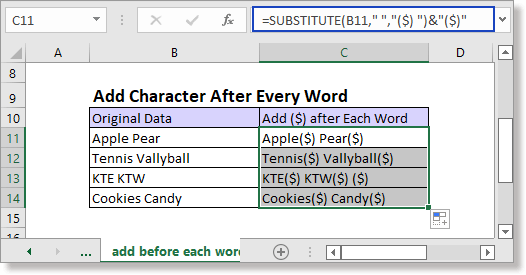6.2 Add character(s) before or after each word by Find and Replace

If you want to add characters before each word excluding the first, or add characters after each word excluding the last as below screenshot shown, the Excel built-in Find and Replace utility can handle this job.

Add before each word excluding first one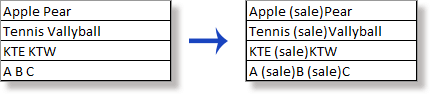Add after each word excluding last one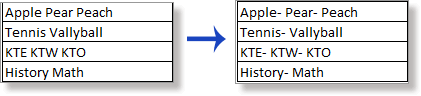1. Select the cells that you want to add characters before or after each word, then press Ctrl + H keys to enable the Find and Replace dialog.

2. Type a space into the Find what textbox, then type the new characters with space in Replace with textbox, and finally click the Replace All button.

 Operation General Example Add characters before each word Add new characters following a space Add (sale) before every word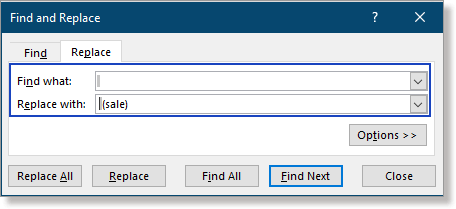Operation General Example Add characters after each word New characters followed with a space Add - after every word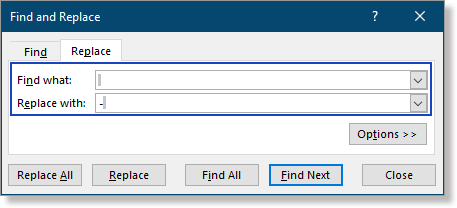#### 6.3 Add character(s) before or after each word by VBA code

Here provides two VBA codes for adding characters before or after each word, please run the code by following below steps.

1. Press Alt + F11 keys to enable the Microsoft Visual Basic for Applications window.

2. In the popping window, click Insert > Module to insert a new blank module.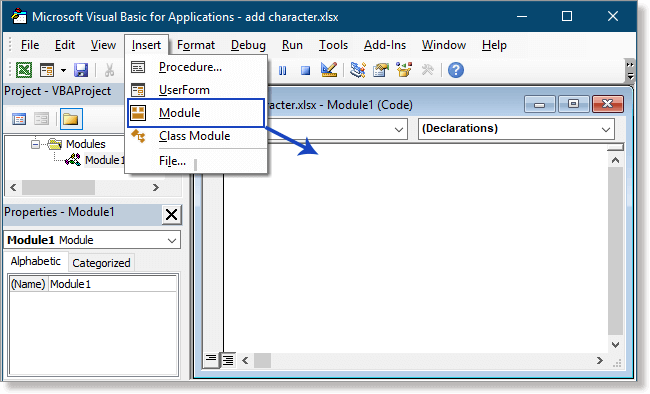3. Copy and paste below VBA code to the module.

``````Sub InsertCharBeforeWord()
'UpdateByExtendOffice
Dim ws As Worksheet
Dim xRg As Range
Dim xSRg As Range
Dim xCell As Range
Dim xInStr As String
Dim xArr As Variant
Dim xValue As String
On Error Resume Next

Set xSRg = Application.Selection
Set xRg = Application.InputBox("Select cells(continuous):", "Kutools for Excel", xSRg.Address, , , , , 8)
If xRg Is Nothing Then Exit Sub
xInStr = Application.InputBox("Type characters you want to add:", "Kutools for Excel", "", , , , , 2)
If StrPtr(xInStr) = 0 Then Exit Sub
Application.ScreenUpdating = False
For Each xCell In xRg
xArr = Split(xCell.Text, " ")
xValue = ""
For Each xStr In xArr
If Trim(xStr) <> "" Then
If xValue = "" Then
xValue = xInStr & Trim(xStr)
Else
xValue = xValue & " " & xInStr & Trim(xStr)
End If
End If
Next
xCell.Value = xValue
Next
Application.ScreenUpdating = True
End Sub``````

``````Sub InsertCharAfterWord()
'UpdateByExtendOffice
Dim ws As Worksheet
Dim xRg As Range
Dim xSRg As Range
Dim xCell As Range
Dim xInStr As String
Dim xArr As Variant
Dim xValue As String
On Error Resume Next

Set xSRg = Application.Selection
Set xRg = Application.InputBox("Select cells(continuous):", "Kutools for Excel", xSRg.Address, , , , , 8)
If xRg Is Nothing Then Exit Sub

xInStr = Application.InputBox("Type characters you want to add:", "Kutools for Excel", "", , , , , 2)
If StrPtr(xInStr) = 0 Then Exit Sub

Application.ScreenUpdating = False
For Each xCell In xRg
xArr = Split(xCell.Text, " ")
xValue = ""
For Each xStr In xArr
If Trim(xStr) <> "" Then
If xValue = "" Then
xValue = Trim(xStr) & xInStr
Else
xValue = xValue & " " & Trim(xStr) & xInStr
End If
End If
Next
xCell.Value = xValue
Next
Application.ScreenUpdating = True
End Sub``````

4. Press F5 key to run the code (or you can click Run button), a dialog pops out to ask you to select continuous cells to work, click OK.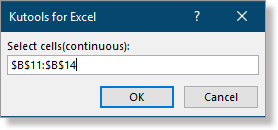5. In the second pops out dialog, type the characters that you want to add, click OK.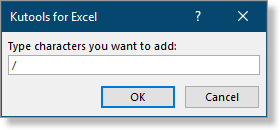Then the characters will be added at the front or end of each word.

#### 7. Add text between characters

Sometimes, you may want to add text between every characters. Here in this part, it lists two scenarios, one is to add text between every character, another one is to add space between each number.

7.1 Add text between every character/digit with User-Defined function

In this case, we add a space between every character.

1. Press Alt + F11 keys to enable the Microsoft Visual Basic for Applications window.

2. In the popping window, click Insert > Module to insert a new blank module.3. Copy and paste below VBA code to the module.

``````Function AddText(Str As String) As String
Dim i As Long
For i = 1 To Len(Str)
Next i
End Function``````

4. Save the code and close the window to go back to the worksheet, then type below formula into a cell that you want to place the adding result.

B3 is the cell contains the string that you want to add space between characters.

5. Press Enter key to get the result, drag auto fill handle over cells to get other results as you need.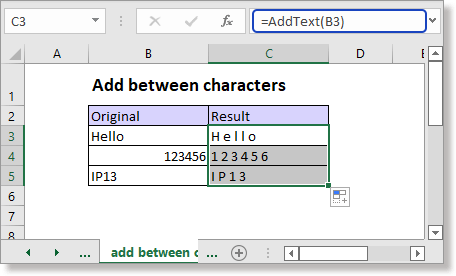Attention: 1） In the VBA code, you can change the " " to other texts as you need, supposing change it to "-" to add - between characters. 2） The code will add the new text after every character. You can remove the last added text if you do not need by using below formula. For more details about the formula, please visit: LEFT(cell,LEN(cell)-text_length)

7.2 Add space between every number with formula

If you want to add space between digits of a string which is a numeric value, you can use the TEXT function.

 Attention: the numbers of range you want to add space between needed to be in the same length, otherwise, some results may be incorrect.

Supposing here is a list of 8-digit numbers in range B10:B12, please select a cell that will place the result, type below formula:

=TEXT(B10,"# # # # # # # #")

Press Enter key, then drag autofill handle over other cells.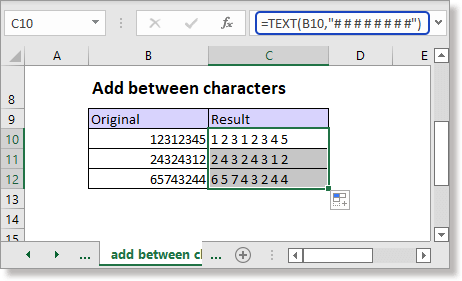If the length of numbers is 5, the formula will be changed as =TEXT(B10,"# # # # #").

#### 8. Add character(s) between every word

If you want to add character(s) between every word, supposing add space between first, middle, last names as below screenshot shown, you can use a User-Defined function.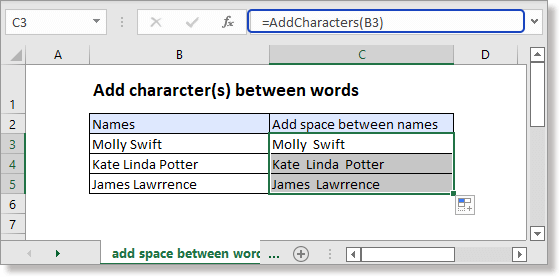1. Press Alt + F11 keys to enable the Microsoft Visual Basic for Applications window.

2. In the popping window, click Insert > Module to insert a new blank module.

3. Copy and paste below VBA code to the module.

``````Function AddCharacters(pValue As String) As String
'UpdatebyExtendoffice20160908
Dim xOut As String
xOut = VBA.Left(pValue, 1)
For i = 2 To VBA.Len(pValue)
xAsc = VBA.Asc(VBA.Mid(pValue, i, 1))
If xAsc >= 65 And xAsc <= 90 Then
xOut = xOut & " " & VBA.Mid(pValue, i, 1)
Else
xOut = xOut & VBA.Mid(pValue, i, 1)
End If
Next
End Function``````

4. Save the code and close the window to go back to the worksheet, then type below formula into a cell that you want to place the adding result.

B3 is the cell contains the string that you want to add space between words.

5. Press Enter key to get the result, drag auto fill handle over cells to get other results as you need.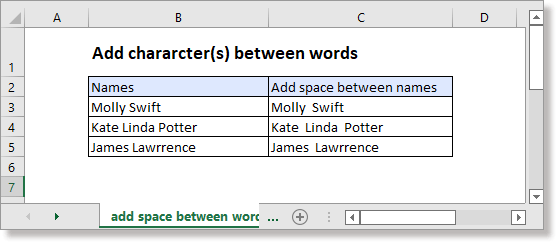Attention: in the VBA code, you can change the " " to other texts as you need, supposing change it to "-" to add - between words.

#### 9. Add character between texts and numbers

To add specific character(s) between texts and numbers as below screenshot shown, here provides two methods.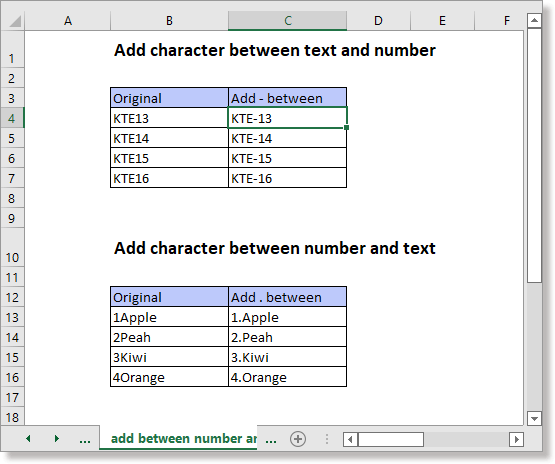9.1 Add character between texts and numbers with formula

If the texts are in the front of the numbers, the generic formula is

TRIM(REPLACE(string,MIN(FIND({1,2,3,4,5,6,7,8,9,0},string&"1234567890")),0,"-"))

Using above case as example, please use the formula as this:

=TRIM(REPLACE(B4,MIN(FIND({1,2,3,4,5,6,7,8,9,0},B4&"1234567890")),0,"-"))

Press Enter key to get the first result, then drag auto fill handle over other cells to fill this formula.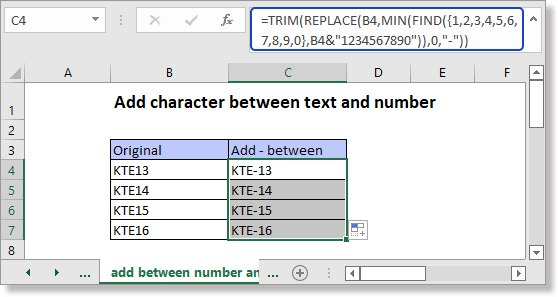If the numbers are in the front of the texts, the generic formula is

LEFT(string,SUM(LEN(string)-LEN(SUBSTITUTE(string,{0,1,2,3,4,5,6,7,8,9},""))))&"."&RIGHT(string,LEN(B13)- SUM(LEN(string)-LEN(SUBSTITUTE(string, {0,1,2,3,4,5,6,7,8,9}, ""))))

Using above case as an example, please use the formula as this:

=LEFT(B13,SUM(LEN(B13)-LEN(SUBSTITUTE(B13,{0,1,2,3,4,5,6,7,8,9},""))))&"."&RIGHT(B13,LEN(B13)- SUM(LEN(B13)-LEN(SUBSTITUTE(B13, {0,1,2,3,4,5,6,7,8,9}, ""))))

Press Enter key to get the first result, then drag auto fill handle over other cells to fill this formula.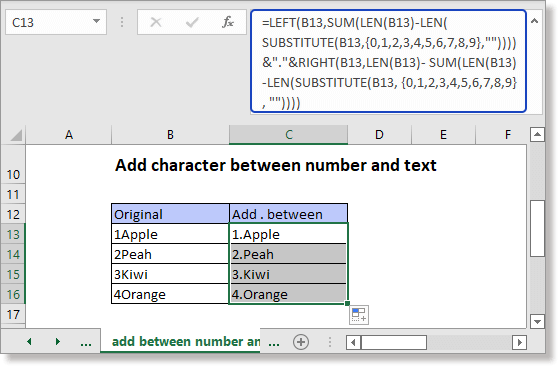9.2 Add character between texts and numbers with Flash Fill

If you are in Excel 2013 or later versions, the Flash Fill also can help you to add character(s) between texts and numbers.

Next to the original data, type the new data which have been added the character(s) between texts and numbers. Then press Enter key to go to the next cell of the first result.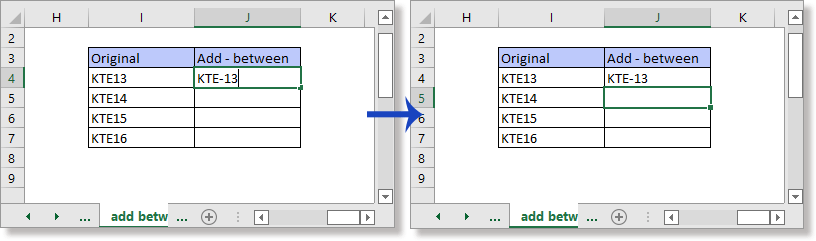And press Ctrl + E keys to enable Flash Fill.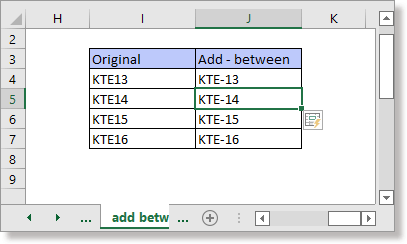Extension

This tutorial also lists some scenarios about adding text that we may meet in our daily life or work.

#### 10. Add dashes to phone numbers/social numbers

Sometimes, you need to add separators such as dashes to a serial of phone numbers or social numbers as below screenshot shown. Manually typing will be tried if there are hundreds of numbers needed to be added. In this part, it introduces three trick ways to handle this job quickly.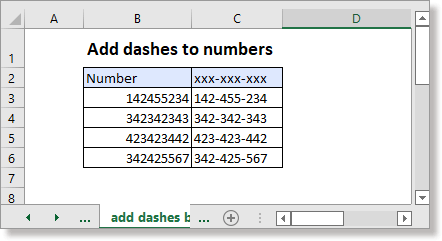10.1 Add dashes to numbers by formula

Here are two formulas can quickly add dashes to numbers. To add dashes after the third and sixth numbers in cell B3, please use the formula as these:

REPLACE function

=REPLACE(REPLACE(B3,4,0,"-"),8,0,"-")

TEXT function

=TEXT(B3,"???-???-??? ")

Press Enter key to get the first result, then drag auto fill handle over other cells.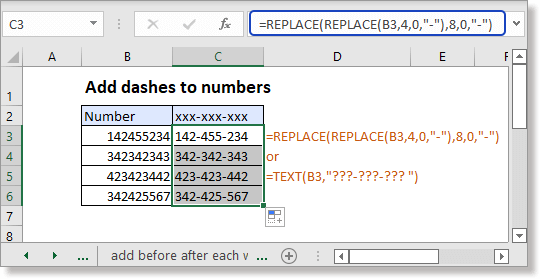Attention: You can change above formulas’ arguments as you need. Supposing add "-" after the second, fourth and sixth digits, you can use the formulas as: =REPLACE(REPLACE(REPLACE(B3,3,0,"-"),6,0,"-"),9,0,"-") =TEXT(B3,"??-??-??-??? ")

10.2 Add dashes to numbers by Format Cells

To directly add dashes to the numbers in the original cells, you can use the Format Cells feature.

1. Select the numbers that you want to add dashes to, then press Ctrl + 1 keys to enable the Format Cells dialog.

2. In the Format Cells dialog, under the Number tab, choose Custom from the Category section.

3. Then in the right Type section, type ###-###-### into the textbox, click OK.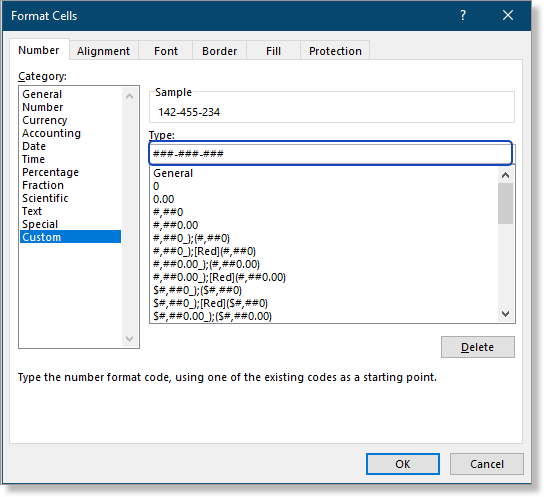Now the selected numbers have been added dashes.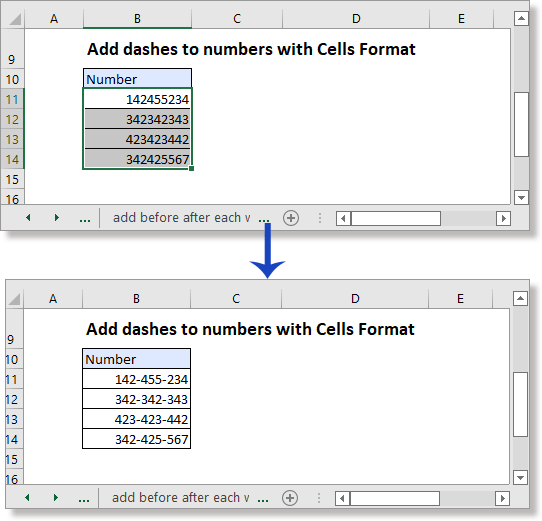10.3 Add dashes to phone number by Add Text of Kutools for Excel

If you have Kutools for Excel installed in Excel, the Add Text feature also can do you a favor.

1. Select the cells that contain the numbers needed to be added dashes, click Kutools > Text > Add Text.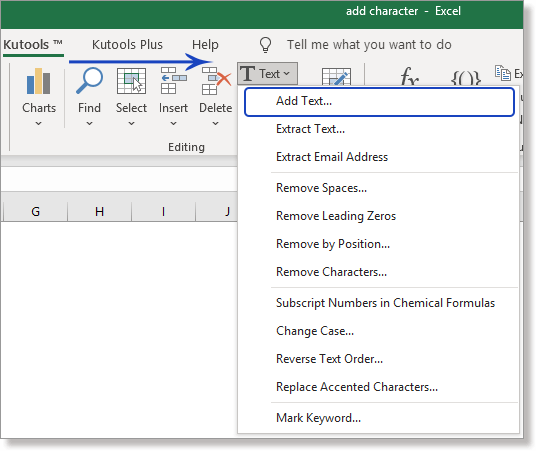2. In the Add Text dialog, type the dash or other separator into Text textbox, choose Specify option, then type the positions you want to add dashes after and separate them by commas into the below textbox.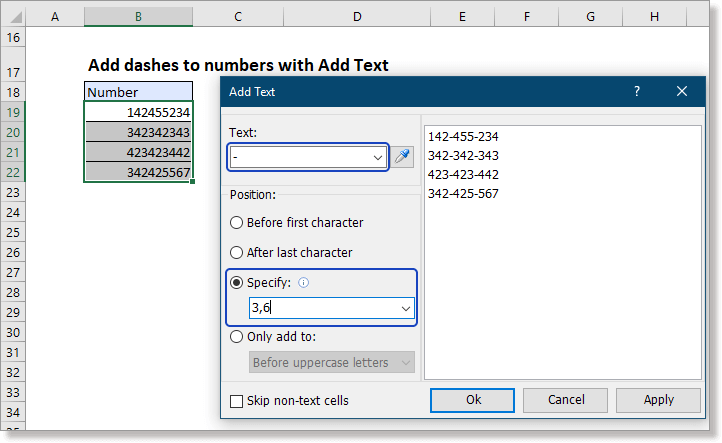3. Click Ok or Apply. Then the selected numbers have been added dashes.

Supposing there is a list of numbers in different lengths, you want to add leading zeros to make them in the same length and look neat as below screenshot shown. Here are two methods provided for you to handle the job.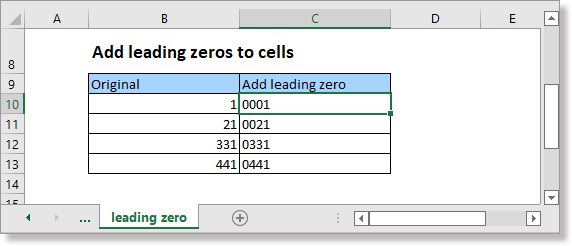You can use the Format Cells feature to set a custom format to fix the cell length by adding leading zeros.

1. Select the cells that you want to add leading zeros, then press Ctrl + 1 keys to enable Format Cells dialog.

2. In the Format Cells dialog, under the Number tab, choose Custom from the Category section.

3. Then in the right Type section, type 0000 into the textbox (to fix the number length to 4-dight, type 0000, you can change it to other length, such as 5-dight, type 00000), click OK.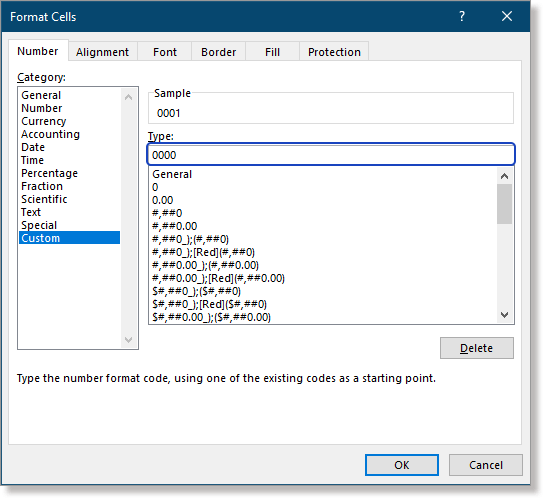Then the numbers have been added leading zeros and in the same length.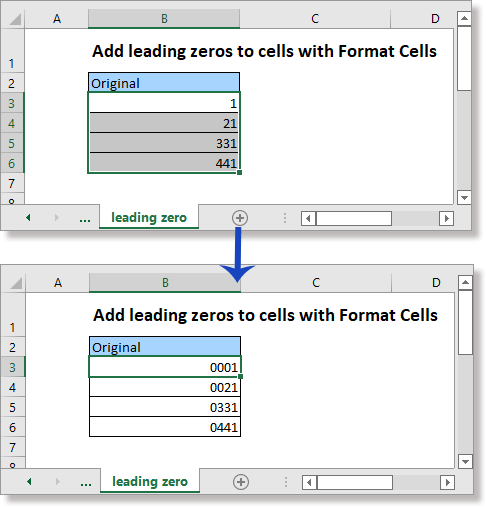Attention: if the length of the original data exceeds the set length, it will display the original data without leading zeros.

If you do not want to change the original data, you can use formula to add leading zeros to numbers in other locations.

Formula1 TEXT function

TEXT(number,"00…")

Formula2 RIGHT function

RIGHT("00…"&number,length)

Formula3 BASE function

BASE(number,10,length)

The number of zeros in the formula is equal to the length of the number.

Here you set 4-digit as the length of the numbers, please use the formulas as below:

=TEXT(B10,"0000")

=RIGHT("0000"&B10,4)

=BASE(B10,10,4)

Press Enter key and drag auto fill handle down.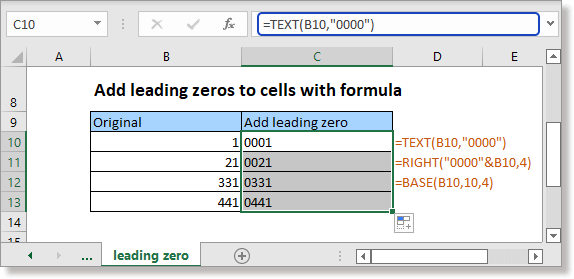Attention: if the length of the original data exceeds the set length, it will display the original data without leading zeros.

#### 12. Add trailing zeros to numbers to fix the length

If you want to add trailing zeros to numbers for making a fixed length, supposing adding trailing zeros to the numbers in cell B3:B7 to make them in 5-digit length as below screenshot shown, you can apply a formula to deal with the job.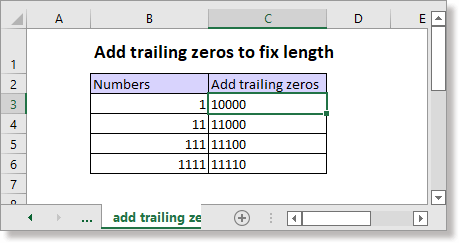REPT function

number&REPT("0",length-LEN(number))

In the formula, the argument "number" represents the number or a cell reference that you want to add trailing zeros, and the argument "length" is the fixed length you want.

Please use the formula as this:

=B3&REPT("0",5-LEN(B3))

Press Enter key and drag auto fill handle down to cells that need this formula.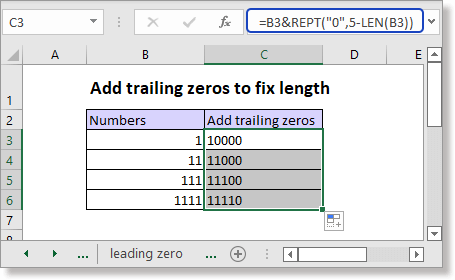#### 13. Add trailing space to text

If you want to export or copy data from a worksheet to a notepad, the texts may be placed untidily as the cell values are in different lengths, for making them look neat as below screenshot shown, you need to add trailing space.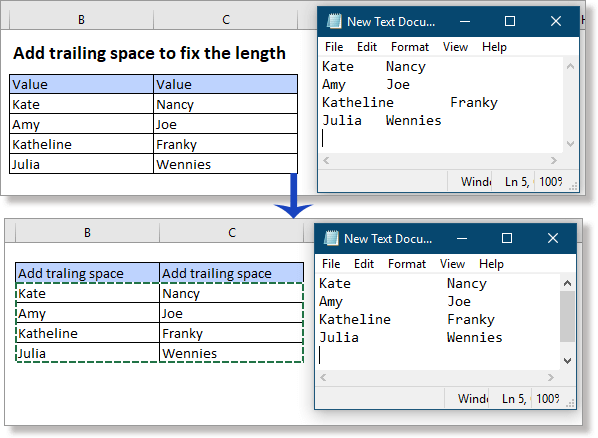For solving this job, the REPT function can do you a favor.

LEFT(text & REPT(" ",max_length),max_length)

In the formula, the argument "text" is the text or the cell reference that you want to add trailing space, and the argument "max_length" is the fixed length you want to make the texts, which must be longer than or equal to the maximum length of the used texts.

To add trailing space to the texts in cell B3:C6, please use below formula:

=LEFT(B3 & REPT(" ",10),10)

Press Enter key, then drag auto fill handle over to all cells needed this formula.

In this case, as the longest of the used text is 9-character, we use 10 here.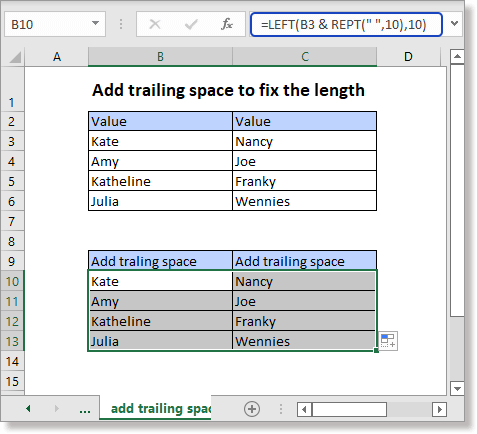#### 14. Add quotation marks/brackets around text or numbers

Sometimes, you need to add quotation marks or brackets around the text or numbers in cells as below screenshot shown, except manual typing one by one, here are two methods in Excel can quickly solve it.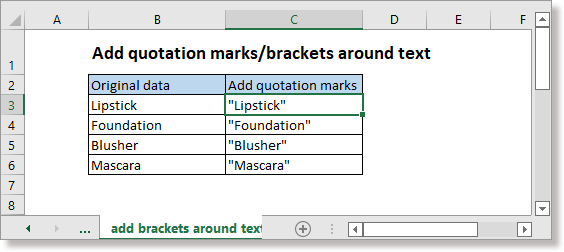14.1 Add quotation marks/brackets around by Format Cells

If you want to add quotation marks around text in the original data, the Format Cells feature in Excel can help you.

1. Select the cells that you want to add quotation marks, press Ctrl + 1 keys to enable the Format Cells dialog.

2. In the Format Cells dialog, under Number tab, choose Custom from the list of Category, then go to right section to type "''"@"''" into the Type textbox. Click OK.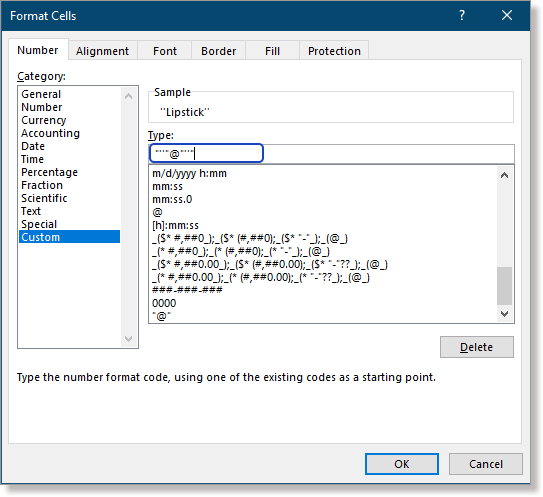The text in selected cells have been added around with quotation marks.

If you want to add brackets around the text, in the Type textbox of the Format Cells dialog, use (@).

14.2 Add quotation marks/brackets around by formulas

Formulas also can be used to add quotation marks to text in other cells.

Using & connector

""""&text&""""

Or

char(34)&text&char(34)

In this case, text in the cell B11, please use the formulas as these:

=""""&B11&""""

Or

=char(34)&B11&char(34)

Press Enter key and drag auto fill handle down to fill cells.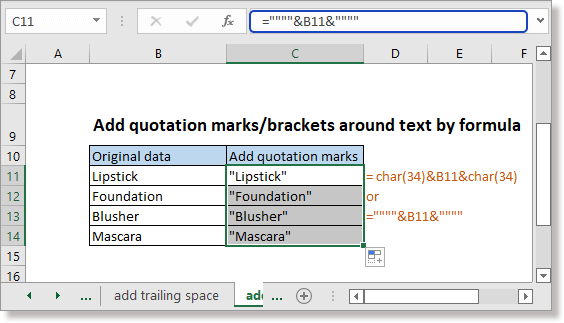If you want to add brackets around text, using the formula as "("&text&")".

14.3 Add quotation marks/brackets around by VBA

If you need VBA code to solve this job, here is a VBA.

1. Press Alt + F11 keys to enable the Microsoft Visual Basic for Applications window.

2. Then in the popping window, click Insert > Module to insert a blank new module.

3. Copy and paste below VBA code to the new module.

VBA: Add quotation marks around texts

``````Sub addquotationmarksorbrackets()
'UpdatebyExtendOffice
Dim Rng As Range
Dim WorkRng As Range
On Error Resume Next
xTitleId = "KutoolsforExcel"
Set WorkRng = Application.Selection
Set WorkRng = Application.InputBox("Range", xTitleId, WorkRng.Address, Type:=8)
For Each Rng In WorkRng
Rng.Value = """" & Rng.Value & """"
Next
End Sub``````
 Attention: in the code script, """" & Rng.Value & """" indicates to add "" around the texts, if you want to add brackets () around text, change it to "(" & Rng.Value & ")".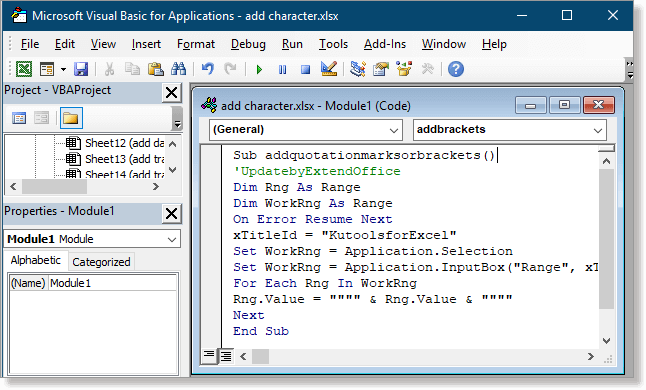4. Press F5 key or click Run buttonin the window to activate the VBA code. A dialog pops out for selecting cells to add quotes.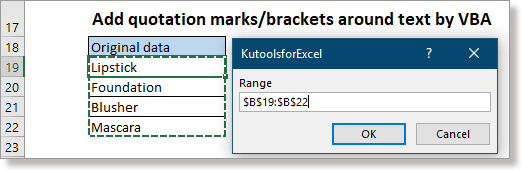5. Click OK. Now the quotation marks have been added around texts in the selected cells.

#### 15. Add text to formula result directly

Sometimes, to help other users better understand data, you may need to add text in the formula results as below screenshot shown. For including text in formula, you can use the quotation marks surrounding the text and add the & connector to combine the text and the formula together.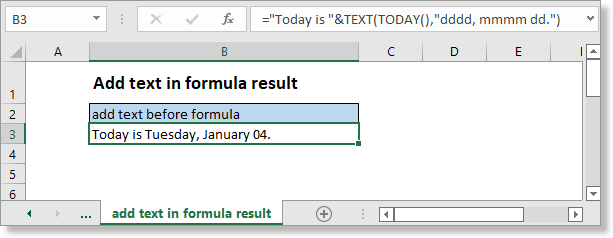Case 1 add text before formula

"Today is "&TEXT(TODAY(),"dddd, mmmm dd.")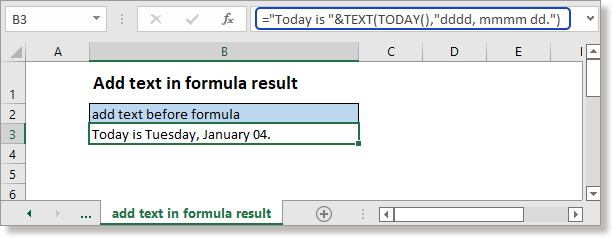Case 2 add text after formula

TEXT(NOW(),"HH:MM:SS")&" is the current time."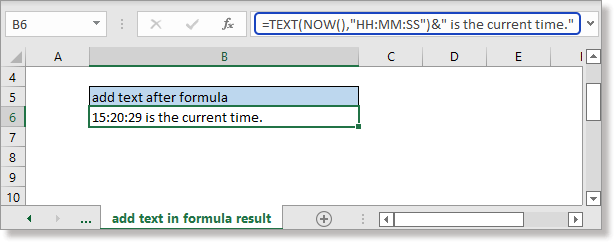Case 3 add texts at two side of formula

"Today sold "&data&" kg."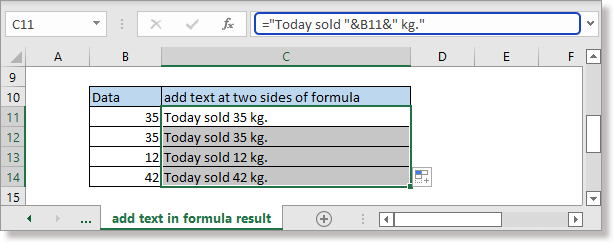#### More Excel Tutorials:

Combine Multiple Workbooks/Worksheets Into One
This tutorial, listing almost all combining scenarios you may face and providing relative professional solutions for you.

Split Text, Number, And Date Cells (Separate Into Multiple Columns)
This tutorial is divided into three parts: split text cells, split number cells and split date cells. Each part provides different examples to help you know how to handle the splitting job when encountering the same problem..

Combine Contents Of Multiple Cells Without Losing Data In Excel
This tutorial narrows down the extraction to a specific position in a cell and collects different methods to help extract text or numbers from a cell by specific position in Excel.

Compare Two Columns For Matches And Differences In Excel

## The Best Office Productivity Tools

### Kutools for Excel Solves Most of Your Problems, and Increases Your Productivity by 80%

• Super Formula Bar (easily edit multiple lines of text and formula); Reading Layout (easily read and edit large numbers of cells); Paste to Filtered Range...
• Merge Cells/Rows/Columns and Keeping Data; Split Cells Content; Combine Duplicate Rows and Sum/Average... Prevent Duplicate Cells; Compare Ranges...
• Select Duplicate or Unique Rows; Select Blank Rows (all cells are empty); Super Find and Fuzzy Find in Many Workbooks; Random Select...
• Exact Copy Multiple Cells without changing formula reference; Auto Create References to Multiple Sheets; Insert Bullets, Check Boxes and more...
• Favorite and Quickly Insert Formulas, Ranges, Charts and Pictures; Encrypt Cells with password; Create Mailing List and send emails...
• Extract Text, Add Text, Remove by Position, Remove Space; Create and Print Paging Subtotals; Convert Between Cells Content and Comments...
• Super Filter (save and apply filter schemes to other sheets); Advanced Sort by month/week/day, frequency and more; Special Filter by bold, italic...
• Combine Workbooks and WorkSheets; Merge Tables based on key columns; Split Data into Multiple Sheets; Batch Convert xls, xlsx and PDF...
• Pivot Table Grouping by week number, day of week and more... Show Unlocked, Locked Cells by different colors; Highlight Cells That Have Formula/Name...### Office Tab - brings tabbed interface to Office, and make your work much easier

• Enable tabbed editing and reading in Word, Excel, PowerPoint, Publisher, Access, Visio and Project.
• Open and create multiple documents in new tabs of the same window, rather than in new windows.
• Increases your productivity by 50%, and reduces hundreds of mouse clicks for you every day!